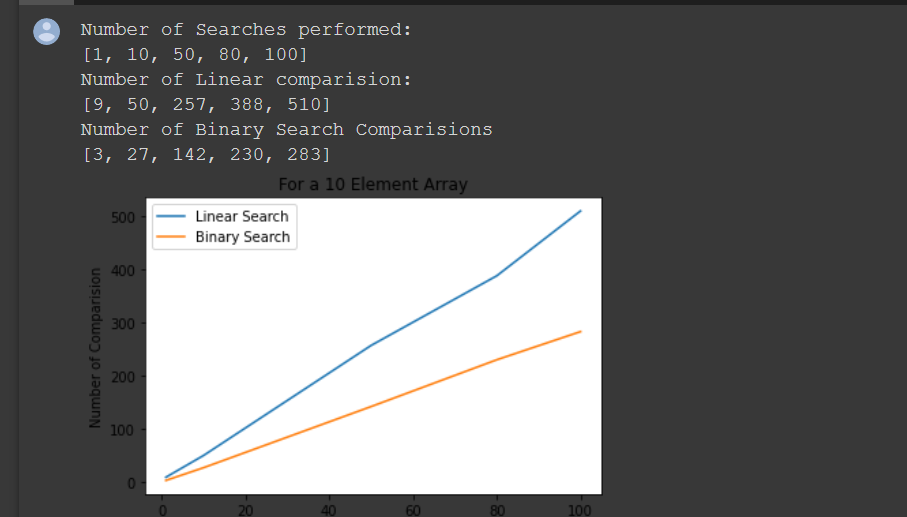# Linear Search vs Binary Search time comparison in Python

In this tutorial, we are going to learn about linear search and binary search in Python. It’ll help us to justify where should we use binary search and where linear search. So we are willing to do this in python and to do this we need matplotlib.

## Installation of matplotlib

If you have already installed matplotlib in your machine then you can look over this step. If you don’t then go to command prompt( For Mac or Linux users open terminal). Then enter the following code and run it.

```python -m pip install -U pip

python -m pip install -U matplotlib

```

## Linear Search vs Binary Search time comparison

At first, you have to write the linear search and binary search code(Note: For binary search if the array isn’t sorted then sort the array):

For Linear search, you can go through to this link: https://codespeedy.com/linear-search-implement-in-python/

For Binary search, ou can go through to this link: https://codespeedy.com/binary-search-in-python-and-how-to-implement/

### Python code:

In python first, you have to import pyplot from matplotlib.

Then you have to take 3 arrays containing three arrays in which we will store the value of comparisons, no searches performed, etc.

Now a ‘for’ loop will run for ‘no of searches’ times to store the values in the array.

The Python code is shown below:

```def draw_plot(number_of_elements):
array = np.random.randint(1,high=100000,size=number_of_elements, dtype=int)

x_axis = []
y_axis = []                                                         # Comparision for Linear Search list
z_axis = []                                                         # Comparision for Binary Search list

number_of_comparison_linear = 0
number_of_comparison_binary = 0

for i in range(1,2):
random_index_for_search = np.random.randint(0,len(array)-1)      # As we have 10k elements we take a random index b/w 0...99999
a = linear_search(array, target=array[random_index_for_search])
number_of_comparison_linear += a["Position"] + 1

b = binary_search(array, target=array[random_index_for_search])
number_of_comparison_binary += b["Comparison"]

x_axis.append(1)

y_axis.append(number_of_comparison_linear)

z_axis.append(number_of_comparison_binary)

number_of_comparison_linear = 0
number_of_comparison_binary = 0

for i in range(1,1001):
random_index_for_search = np.random.randint(0,len(array)-1)      # As we have 10k elements we take a random index b/w 0...99999
a = linear_search(array, target=array[random_index_for_search])
number_of_comparison_linear += a["Position"] + 1

b = binary_search(array, target=array[random_index_for_search])
number_of_comparison_binary += b["Comparison"]

x_axis.append(1000)

y_axis.append(number_of_comparison_linear)

z_axis.append(number_of_comparison_binary)

number_of_comparison_linear = 0
number_of_comparison_binary = 0

for i in range(1,5001):
random_index_for_search = np.random.randint(0,len(array)-1)      # As we have 10k elements we take a random index b/w 0...99999
a = linear_search(array, target=array[random_index_for_search])
number_of_comparison_linear += a["Position"] + 1
b = binary_search(array, target=array[random_index_for_search])
number_of_comparison_binary += b["Comparison"]

x_axis.append(5000)
y_axis.append(number_of_comparison_linear)

z_axis.append(number_of_comparison_binary)

number_of_comparison_linear = 0
number_of_comparison_binary = 0

for i in range(1,8001):
random_index_for_search = np.random.randint(0,len(array)-1)      # As we have 10k elements we take a random index b/w 0...99999
a = linear_search(array, target=array[random_index_for_search])
number_of_comparison_linear += a["Position"] + 1

b = binary_search(array, target=array[random_index_for_search])
number_of_comparison_binary += b["Comparison"]

x_axis.append(8000)
y_axis.append(number_of_comparison_linear)

z_axis.append(number_of_comparison_binary)

number_of_comparison_linear = 0
number_of_comparison_binary = 0

for i in range(1,10001):
random_index_for_search = np.random.randint(0,len(array)-1)      # As we have 10k elements we take a random index b/w 0...99999
a = linear_search(array, target=array[random_index_for_search])
number_of_comparison_linear += a["Position"] + 1

b = binary_search(array, target=array[random_index_for_search])
number_of_comparison_binary += b["Comparison"]

x_axis.append(10000)
y_axis.append(number_of_comparison_linear)

z_axis.append(number_of_comparison_binary)

print("Number of Searches performed:")
print(x_axis)
print("Number of Linear comparision:")
print(y_axis)
print("Number of Binary Search Comparisions")
print(z_axis)

plt.plot(x_axis, y_axis)
plt.plot(x_axis, z_axis)
plt.title("For a " + str(number_of_elements) + " Element Array")
plt.xlabel("Number of Searches performed")
plt.ylabel("Number of Comparision")
plt.legend(["Linear Search", "Binary Search"])
plt.show()```

I’m also giving you the whole code link, in which you can get the whole code:

comparson.py

Open the zip file to get the .py file.

You may show the outputs like this: